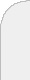inicioir al DIIbuscarprimal and convex hull relaxations for nonlinear integer programming problems  Obtaining strong bounds for nonlinear integer programming problems has always been a challenge. Lagrangean relaxation is usually not appropriate, since the subproblems are still nonlinear integer problems, and unlikely to be easier to solve than the original problem. Relaxations based on solving linear integer problems are more likely to be successful.The primal relaxation introduced by Guignard in 1994 relies on solving linear integer programming problems over convex hulls of integer solutions feasible for a subset of the constraints. Solution algorithms are based on penalty or augmented Lagrangean methods and either Frank and Wolfe or simplicial decomposition. They have been studied by Contesse and Guignard and implemented by Ahn, Ahlatcioglu and Guignard. When all constraints are kept in the subproblems, one obtains the so-called convex hull relaxation or CHR (Albornoz, independently rediscovered by Ahlatcioglu). This relaxation will only work if one can solve relatively quickly linear counterparts of the original nonlinear integer problems. If these linear problems have the integrality property, one will only obtain the continuous relaxation of the original problem.Since both primal and convex hull relaxations rely on Frank and Wolfe or on simplicial decomposition, convergence of the process to a valid bound requires convexity of the objective function. If the function is nonconvex but quadratic, and the variables are all 0-1, convexification is possible via SDP optimization (Billionnet, Elloumi and Plateau for instance). The bounds obtained via convexification have so far been disappointing; however they allow the otherwise impossible solution of moderately sized problems via cplex. An advantage of CHR is that all constraints remain in the subproblems, thus all integer solutions found during the algorithm are feasible. This makes CHR an attractive primal heuristic. In particular it can be used for nonconvex as well as convex problems.We will present results for several types of quadratic assignment problems, both convex and nonconvex.